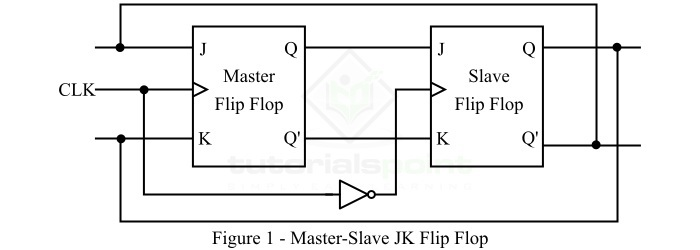# Operation of J-K Master-Slave Flip-Flop

In a normal JK flip flop, there is a problem of race around condition when both inputs, i.e. J and K are equal to 1. To overcome this problem, a modified version of JK flip flop is developed which is called master-slave JK flip flop. In other words, the problem of race around is avoided by operating the JK flop in master and slave modes.

The Master-Slave J-K Flip Flop is basically a combination of two JK flip flops which are connected in the series manner as shown in the following figure.In the series combination of two JK flip flops as shown in above figure, one flip flop acts as a master flip flop and the other one acts as a slave flip flop. In the case of master-slave flip flop, the outputs of the master JK flip flop are connected to the inputs of the slave JK flip flop. The outputs of the slave flip flop are fed back to the inputs of the master JK flip flop as shown in the circuit diagram.

In the master-slave JK flip flop, an inverter gate is used which is connected to clock in a way that the inverted clock pulse is applied to the slave flip flop and the normal clock pulse is applied to the master flip flop. Consequently, when clock pulse to master flip flop is 0, then for slave flip flop, the clock pulse is 1, and if the clock pulse to the master flip flop is 1, then for the slave flip flop it is 0.

After getting insights about JK master-slave flip flop, let us now discuss its operation.

## Operation of J-K Master-Slave Flip-Flop

The J-K Master-Slave Flip-Flop circuit shown in the above figure will operate as described below −

From the logic circuit, it is clear that the master flip flop is positive level triggered and the slave flip flop is negative level triggered. Consequently, the master flip flop responds before the slave flip flop. Which means, when the clock pulse goes to high, the master flip-flop becomes active and the slave flip flop becomes inactive. Thus, the inputs J and K can control the state of the master JK flip flop.

On the other hand, when the clock pulse goes back to low, the information is transferred from master flip flop to the slave flip-flop, and the final output of the master-slave J-K flip-flop is obtained as the output of the slave flip-flop.

Now, let us discuss the operation of the J-K Master-Slave Flip-Flop for different combinations of input values of J and K −

• When J = 0 and K = 0, then both master and slave JK flip flops remain inactive and thus the output Q remains unchanged. This is called Hold State of the master-slave JK flip-flop.

• When J = 0 and K = 1, the output Q’ of the master JK flip flop is high and goes to the input K of the slave JK flip-flop. The clock pulse forces slave JK flip flop to reset. As a result, the slave flip flop have the same output as the master JK flip-flop, i.e. Q’ is high and Q is low. This is called Reset State of the master-slave JK flip flop.

• When J = 1 and K = 0, the output Q of the master JK flip-flop is high and goes to the input J of the slave JK flip-flop, the negative transition of the clock signal sets the slave flip-flop. This is called the Set State of the master-slave JK flip flop.

• When J = 1 and K = 1, the master flip-flop toggles on the positive transition of the clock signal and the slave flip-flop toggles its current state on the negative transition of the clock pulse. Hence, both master and slave flip-flops do not try to toggle simultaneously. Therefore, there is no problem of race around condition in the masterslave JK flip-flop.

We can summarize the operation of the J-K Master-Slave flip flop in the form of a truth table which is given below.

Inputs Output Comment
J K Qn+1
00QnNo Change
010Reset
101Set
11QnToggle

Here, Qn is the current state, and Qn+1 is the next state. In conclusion, the JK master-slave flip-flop is mainly implemented to avoid the problem of race around condition in the JK flipflop. This is all about the operation of J-K Master-Slave Flip-Flop.

Updated on: 24-Apr-2023

837 Views